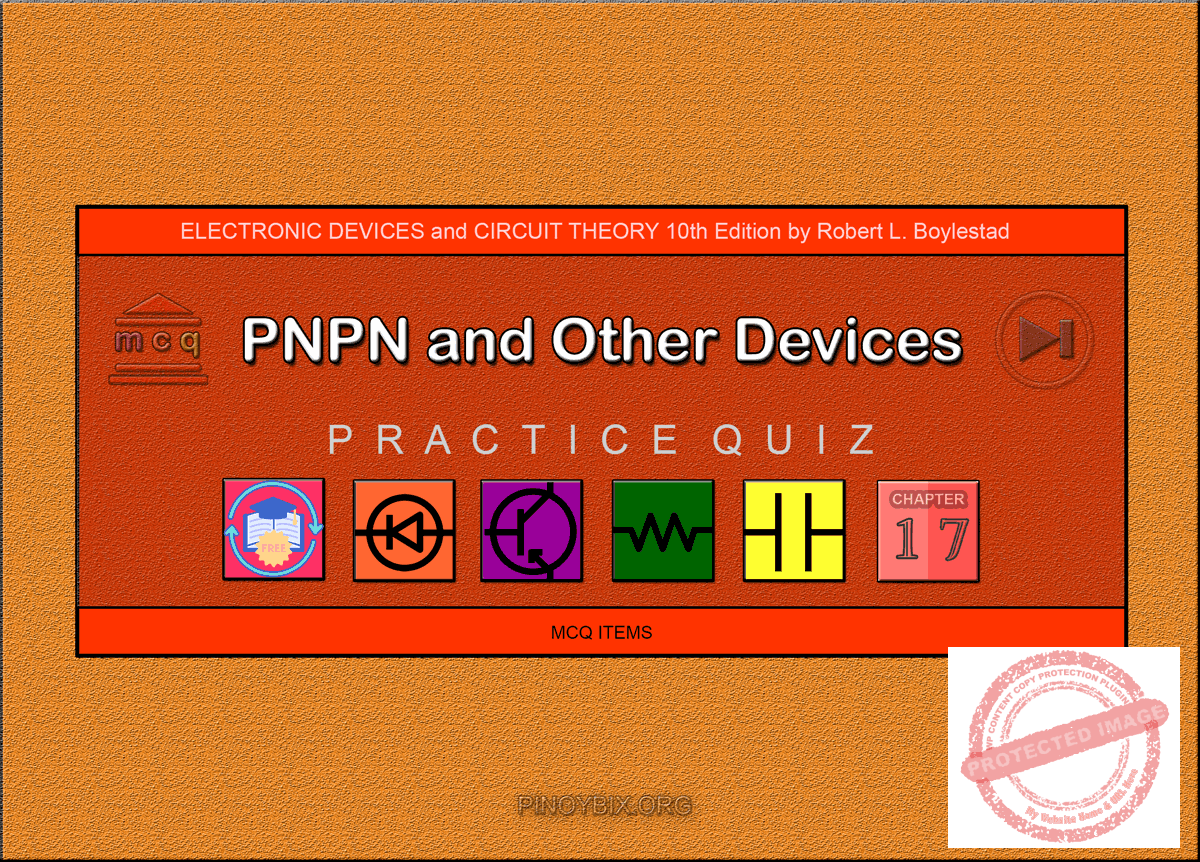(Last Updated On: February 4, 2020)Below are the answers key for the Multiple Choice Questions (Quiz) in pnpn and Other Devices from the book Electronic Devices and Circuit Theory 10th Edition by Robert L. Boylestad.

1. C. 4

2. D. All of the above

3. C. 4

4. A. SCR

6. C. Gate

7. D. 100 kΩ or more

8. A. npn, pnp

9. C. Both npn and pnp

10. B. 10 µs to 25 µs

11. A. 5 µs to 30 µs

12. A. SCR

13. D. All of the above

14. C. 4

15. A. 1.5 mA

16. D. All of the above

17. D. All of the above

18. B. SCS

19. A. negative, positive

20. B. False

21. B. GTO

22. B. GTO

23. C. GTO

24. A. 3 A

25. D. 2

26. D. DIAC

27. C. Triac

28. B. False

29. C. Unijunction transistor

30. A. 50 Ω to 5 kΩ

31. C. AND

32. A. 4

33. B. 3

34. B. 16.25 kΩ

#### FILL-IN-THE-BLANKS

1. D. All of the above

2. A. thyristors

3. D. All of the above

4. C. 10 MW, 2000 A, 1800 V

5. B. anode, gate, and cathode

6. A. 0.01 Ω, 0.1 Ω

7. A. 0.1 μs to 1 μs

8. C. both current interruption and forced commutation

9. D. 100 mA, 20 mA

10. B. 90°

11. A. anode, anode gate, cathode gate, and cathode

12. C. higher, lower

13. D. either on or off

14. B. negative, positive

15. A. larger than

16. C. diac

17. D. All of the above

18. B. base

19. A. An increase, an increase

20. D. All of the above

21. C. both an infrared LED and a photodetector

22. B. decreases

23. D. All of the above

24. A. lower than

25. C. higher than